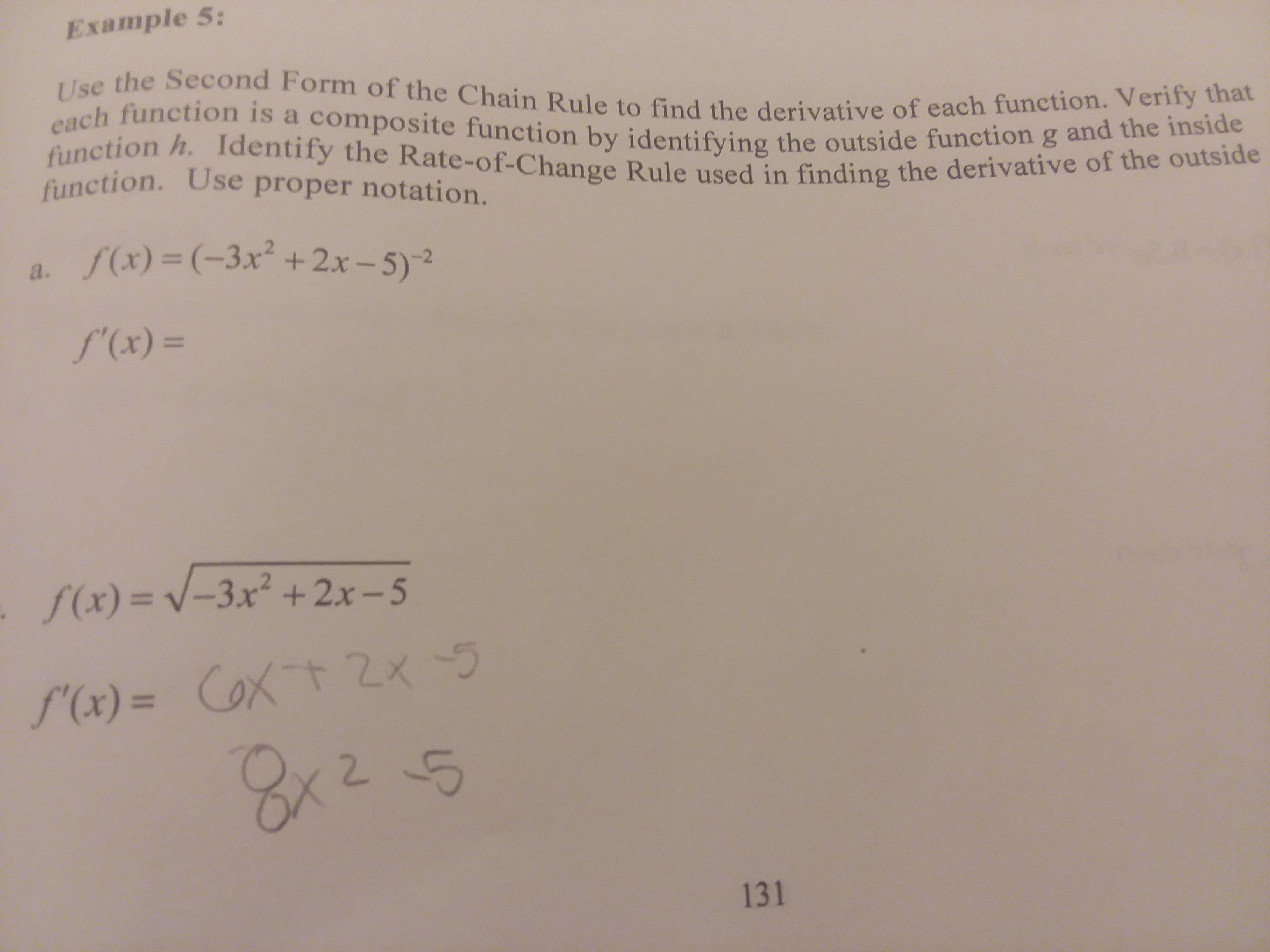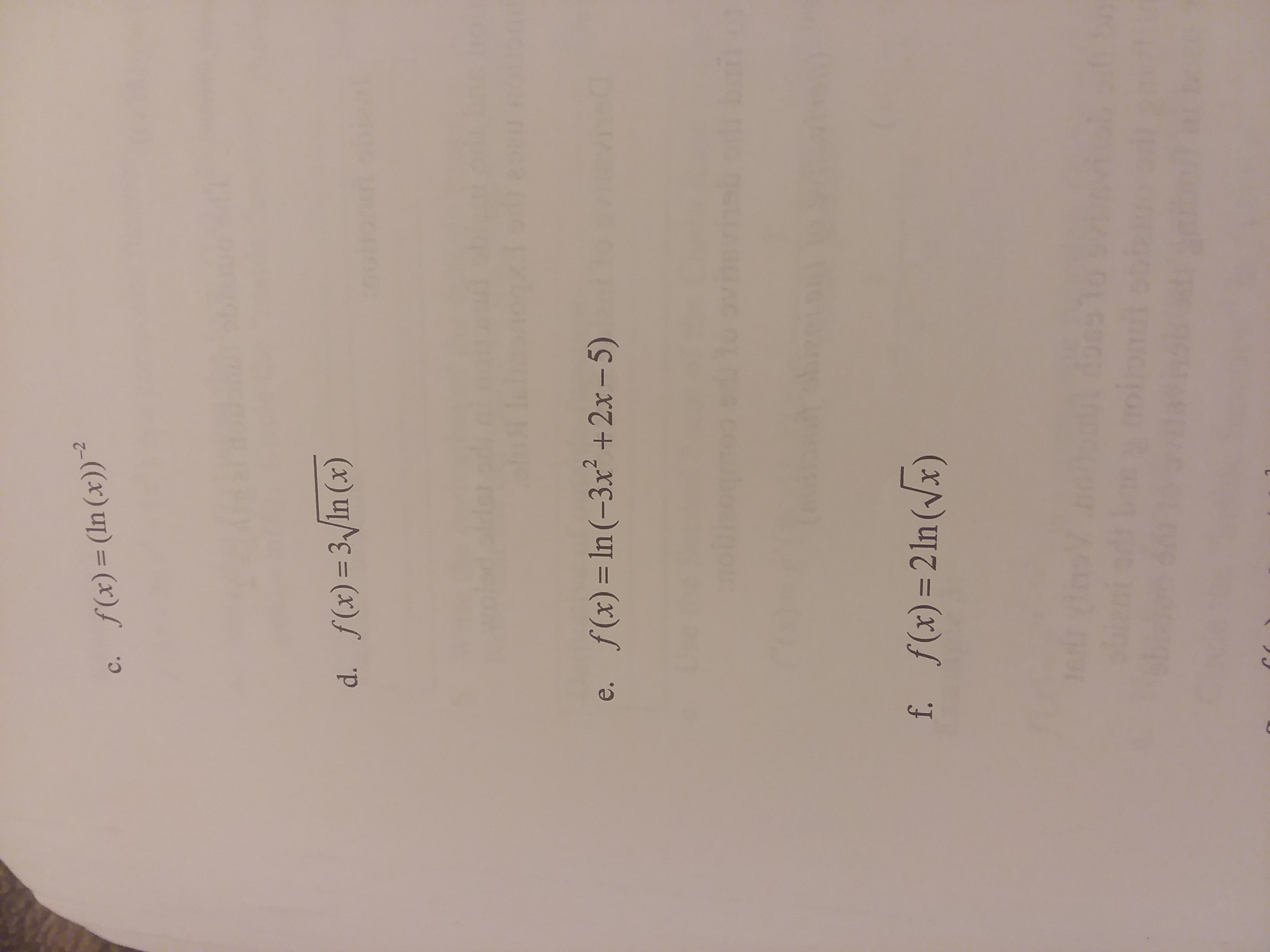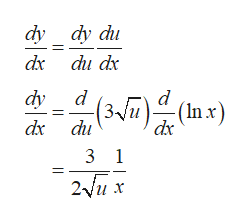# Example 5:Use the Second Form of the Chain Rule to find the derivative of each function. Verify thateach function is a composite function by identifying the outside function g and the insidefunction h. Identify the Rate-of-Change Rule used in finding the derivative of the outsidefunction. Use proper notation.a. f(x)= (-3x +2x-5)-2f'(x) =f(x) = V -3x +2x -5S'(x)= CoXt 2x2 5CoxtZx131 -2f(x) = (In (x))с.d. f(x) 3/In (x)f(x) = In (-3x2 +2x-5)е.f. f(x) 2ln (x)

Question
5 views

I need help with e. and d.

JUST THESE PARTShelp_outlineImage TranscriptioncloseExample 5: Use the Second Form of the Chain Rule to find the derivative of each function. Verify that each function is a composite function by identifying the outside function g and the inside function h. Identify the Rate-of-Change Rule used in finding the derivative of the outside function. Use proper notation. a. f(x)= (-3x +2x-5)-2 f'(x) = f(x) = V -3x +2x -5 S'(x)= CoXt 2x 2 5 CoxtZx 131 fullscreenhelp_outlineImage Transcriptionclose-2 f(x) = (In (x)) с. d. f(x) 3/In (x) f(x) = In (-3x2 +2x-5) е. f. f(x) 2ln (x) fullscreen
check_circle

Step 1

(d)

Consider the given function:

Step 2

Here, let

Step 3

So, f(x) is a composition function that can be written as:

f(x)=g(h(x))=(g o h)(x)

Now,...help_outlineImage Transcriptionclosedy dy du dx du dx dy d d (3Vu (3Jm)(In x) Inx) ( dx du 3 1 2ux fullscreen

### Want to see the full answer?

See Solution

#### Want to see this answer and more?

Solutions are written by subject experts who are available 24/7. Questions are typically answered within 1 hour.*

See Solution
*Response times may vary by subject and question.
Tagged in

### Derivative